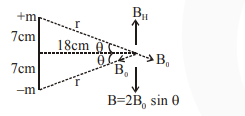# A bar magnet of length 14 cm is placed in the magnetic meridian with its north pole pointing towards the geographic north pole.

Question:

A bar magnet of length $14 \mathrm{~cm}$ is placed in the magnetic meridian with its north pole pointing towards the geographic north pole. A neutral point is obtained at a distance of $18 \mathrm{~cm}$ from the center of the magnet. If $\mathrm{B}_{\mathrm{H}}=0.4 \mathrm{G}$, the

1. $\left.\mathrm{G}=10^{-4} \mathrm{~T}\right)$

2. $2.880 \times 10^{2} \mathrm{~J} \mathrm{~T}^{-1}$

3. $2.880 \mathrm{~J} \mathrm{~T}^{-1}$

4. $28.80 \mathrm{~J} \mathrm{~T}^{-1}$

Correct Option: , 3

Solution:i.e. $\frac{2 \mu_{0}}{4 \pi} \frac{\mathrm{m}}{\mathrm{r}^{2}} \times \frac{7}{\mathrm{r}}=0.4 \times 10^{-4}$

$\Rightarrow 2 \times 10^{-7} \times \frac{\mathrm{m} \times 7}{\left(7^{2}+18^{2}\right)^{3 / 2}} \times 10^{4}$

$=0.4 \times 10^{-4}$

$m=\frac{4 \times 10^{-2} \times(373)^{3 / 2}}{14}$

$M=m \times 14 \mathrm{~cm}=m \times \frac{14}{100}$

$=\frac{0.04 \times(373)^{3 / 2}}{14} \times \frac{14}{100}$

$=4 \times 10^{-4} \times 7203.82=2.88 \mathrm{~J} / \mathrm{T}$